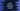# Swift dictionary allSatisfy method - check if all elements satisfy a given predicate## Swift dictionary allSatisfy method :

allSatisfy method is used to check if a predicate is satisfied by all elements in a swift dictionary. This method is defined as below :

``func allSatisfy(((key: Key, value: Value)) -> Bool) -> Bool``

This method takes one predicate and returns one boolean value based on each element satisfies that predicate or not.

### Example of allSatisfy : Check if all values of a dictionary is even :

Let’s consider the below example program :

``````var number_dict = ["one": 1, "two": 2, "three": 3, "four": 4, "five": 5]

func isEvenValue(key: String, value: Int) -> Bool {
return value%2 == 0 ;
}

print(number_dict.allSatisfy(isEvenValue))``````

This program checks if all values of dictionary numberdict_ is even or not. It will print false.

It will print true for the below program :

``````var number_dict = ["two": 2, "four": 4]

func isEvenValue(key: String, value: Int) -> Bool {
return value%2 == 0 ;
}

print(number_dict.allSatisfy(isEvenValue))``````